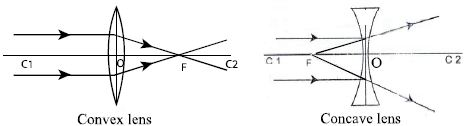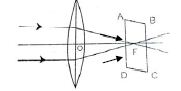# Explain Principal Focus

Principal Focus: When a pencil of parallel rays adjacent to the principal axis incident on a lens in a direction parallel to principal axis, then after refraction the refracted rays either actually converge to a point on the principal axis (in the case of convex lens) or they appear to diverge from a point on the principal axis (in the case of concave lens). This point on the principal axis is called the principal focus of the lens. In figure, the point F is the principal focus of the lens.

Focal length: The distance of the principal focus from the optical centre of a lens is called the focal length of the lens. Focal length is expressed by f. In the figure, OF is the focal length of the lens.Focal plane: The imaginary plane at the principal focus perpendicular to the principal axis is called focal plane of the lens. In the figure, ABCD is the focal plane of the lens.